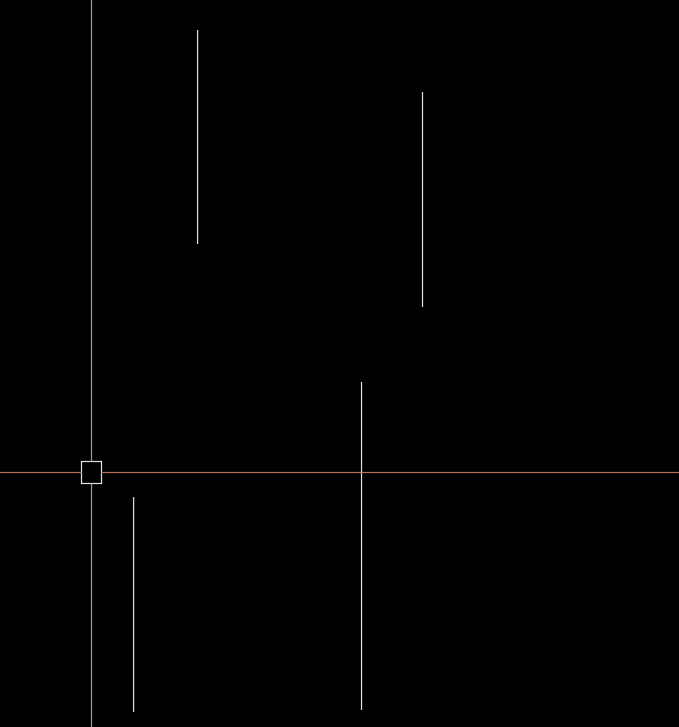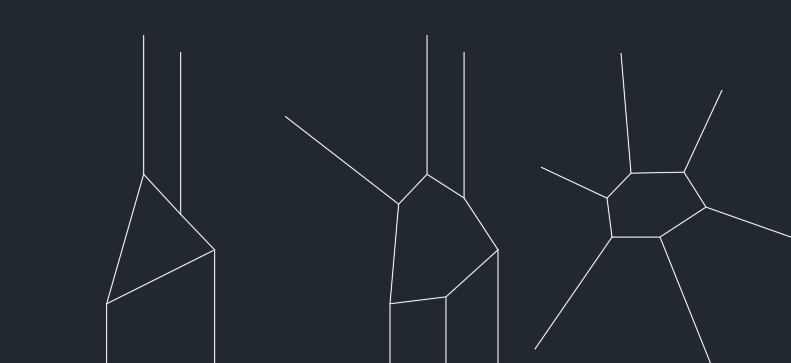# select the 4 points on each line and entmake pline## Recommended PostsCan someone help me to find the solution for some doubts.

If someone will make one lisp for this, so I think I will get answer for few doubts

I need to draw a pline  inside this 4 line using line pointlike this

My doubts

1                     how to get “line point” with window selection (4 line all pints not in window only 4 line 4 point)

```(setq p1 (getpoint "\nSelect object..."))
(setq p2 (getcorner p1))
(setq mp (polar p1 (angle p1 p2) (/ (distance p1 p2) 2)))
(setq p1 (list (nth 0 p1) (nth 1 p1)))
(setq p2 (list (nth 0 p2) (nth 1 p2)))
(if (not (equal '(nil nil) (sssetfirst nil (ssget "_C" p1 p2 '((0 . "LINE")))))) (setq lines (ssget "_:L")))
(if (/= (sslength lines) 4)
(alert "4 lines need to be selected")```

2                     how to segregate the entity (which one is first which one is last)

3                  how to entmake pline

##### Share on other sitesI think select 4 lines then pick a point in approx middle this is needed to make sure get correct end of line else may go to wrong end, the get a list of points sort on angle of point to end of line then draw pline, no idea if it will work just an idea. Need time, should work for any amount say 3+

Edited by BIGAL
##### Share on other sitesMy idea is you can use ssnamex to get the details. As OP says, you select by using crossing window. ssnamex returns details on how the object was added into the selection set. You can then use some sort of formula to determine which point resides within that crossing window... much like using the STRETCH command.

The challenging part is if the selection was made by using crossing lasso.

##### Share on other sitesTry this must be lines for now.

```; https://www.cadtutor.net/forum/topic/70902-select-the-4-points-on-each-line-and-entmake-pline/
; Join end of lines with a pline
; By Alan H July 2020

(defun  AH:joinends ( / pt1 start end d1 d2 temp x ss)
(setq oldsnap (getvar 'osmode))
(setvar 'osmode 0)

(while (setq pt1 (getpoint "Pick point approx middle of line ends Enter to exit"))
(if (setq ss (ssget '((0 . "Line"))))
(progn
(setq lst '())
(repeat (setq x (sslength ss))
(setq obj (vlax-ename->vla-object (ssname ss (setq x (- x 1)))))
(setq end (vlax-get Obj 'Endpoint))
(setq start (vlax-get Obj 'StartPoint))
(setq d1 (distance pt1 end))
(setq d2 (distance pt1 start))
(if (< d1 d2)
(progn
(setq temp end)
(setq end start)
(setq start temp)
)
)
(setq ang (angle pt1 start))
(setq lst (cons (list ang start) lst))
)
(setq lst (vl-sort lst '(lambda (x y) (< (car x)(car y)))))
(command "_pline")
(while (= (getvar "cmdactive") 1 )
(repeat (setq x (length lst))
(command (cadr (nth (setq x (- x 1)) lst)))
)
(command "c")
)
)
)
)

(setvar 'osmode oldsnap)
(princ)
)

(AH:joinends)```##### Share on other sitesHere's another for fun```(defun c:foo (/ a b c p p1 p2 r s)
;; RJP » 2020-07-23
(cond
((and (setq p1 (getpoint "\nPick first corner: "))
(setq p2 (getcorner p1 "\nPick second corner: "))
(setq s (ssget "_C" p1 p2 '((0 . "line,lwpolyline"))))
)
(setq a (vl-sort (mapcar 'car (list p1 p2)) '<))
(setq b (vl-sort (mapcar 'cadr (list p1 p2)) '<))
(foreach e	(vl-remove-if 'listp (mapcar 'cadr (ssnamex s)))
(and (setq p (vl-remove-if-not
'(lambda (x)
(and (<= 10 (car x) 11)
)
)
(entget e)
)
)
(setq r (cons (mapcar 'cdr p) r))
)
)
(if (< 1 (length (setq r (apply 'append r))))
(progn (setq c (mapcar (function (lambda (x) (/ x (length r)))) (apply 'mapcar (cons '+ r))))
(setq r (vl-sort r '(lambda (r j) (< (angle c r) (angle c j)))))
(entmakex	(apply 'append
(list (list '(0 . "LWPOLYLINE")
'(100 . "AcDbEntity")
'(8 . "Closed")
'(100 . "AcDbPolyline")
(cons 90 (length r))
'(70 . 1)
)
(mapcar '(lambda (x) (list 10 (car x) (cadr x))) r)
)
)
)
)
)
)
)
(princ)
)```

Edited by ronjonp
##### Share on other sites@ronjonp nice routine.You may need to check the following before creating the polyline.

`(< 1 (length r))`

##### Share on other sites4 minutes ago, Tharwat said:

@ronjonp nice routine.You may need to check the following before creating the polyline.

```
(< 1 (length r))```

@Tharwat Good idea .. I corrected above to check that there are at least 2 points.

##### Share on other sites
• 1 year later...Let me consider these lines are only 4

From p1 to p2 I need to segregate to inner object and outer object

## Join the conversation

You can post now and register later. If you have an account, sign in now to post with your account.
Note: Your post will require moderator approval before it will be visible.×   Pasted as rich text.   Restore formatting

Only 75 emoji are allowed.